Learn how to derive and use the bilinear transform to convert analog systems into digital ones.

To design and implement a parametric filter, 4 steps are needed:

1. Decide on the filter type you want to implement.
2. Design an analog prototype.
3. Digitize the analog prototype using the bilinear transform.
4. Implement the digital filter in code.

In this article, we’ll explain the second step of that process: how to go from an analog prototype to a digital form of the parametric filter. This is shown on the diagram below.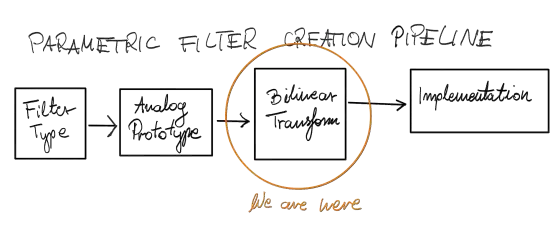Figure 1. In this article, we discuss the bilinear transform.

## System Digitization Methods

There are many methods of digitalization (discretization) of analog (continuous) systems. The most popular ones are [OppenheimSchafer10]:

• impulse-invariant transformation (IIT): sampling the impulse response of the continuous system,
• bilinear transformation (BT): mapping the transfer function of the continuous system from the $s$-plane to the $z$-plane.

Because the impulse-invariant transformation may introduce aliasing, in the music domain, we usually use the bilinear transform. So how is it defined?

## The Bilinear Transform

The bilinear transform is a change of variables, mapping the $j \omega$ frequency axis of the $s$-plane to the unit circle of the $z$-plane.

Given an analog transfer function $H_\text{a}(s)$ in the $s$-domain, we obtain the discrete transfer function $H_\text{d}(z)$ in the $z$-domain by substituting

$s = \frac{2}{T} \frac{1 - z^{-1}}{1 + z^{-1}}, \quad (1)$

where $T = 1 / f_s$ is the sampling interval of the discrete system with $f_s$ being the sampling rate. Therefore,

$H_\text{d}(z) = H_\text{a}\left(\frac{2}{T} \frac{1 - z^{-1}}{1 + z^{-1}}\right). \quad (2)$

We can go back from the discrete transfer function to the analog transfer function by substituting

$z = \frac{1-sT/2}{1+sT/2}, \quad (3)$

what can be directly derived from Equation 1.

The bilinear transform formulas are summarized in Figure 2 (with $c=2/T$).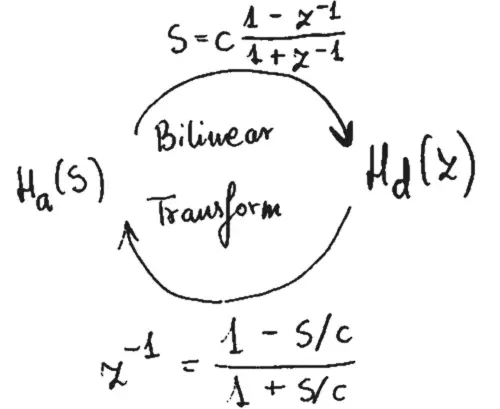Figure 2. Bilinear transform formulas.

## Derivation of the Bilinear Transform

How is the bilinear transform derived? How to come up with the mapping in Equation 1?

Below, I give you a simple, intuitive explanation. If you are not interested in it, you can skip to the properties of the bilinear transform.

The $s \rightarrow z$ mapping of the bilinear transform may be explained as a derivation of a digital representation of an integrator.

An integrator is a system at whose output the integral of the input signal may be observed

$y(t) = \int \limits_0^t x(\tau) d\tau, \quad (4)$

where $x(t)$ is the input signal over continuous time $t$ and $y(t)$ is the output signal of the integrator.

The Laplace transform of Equation 4 yields

$Y(s) = \frac{1}{s}X(s), \quad (5)$

where $s \in \mathbb{C}$. Therefore, the integrator can be shown on the diagram as in Figure 3.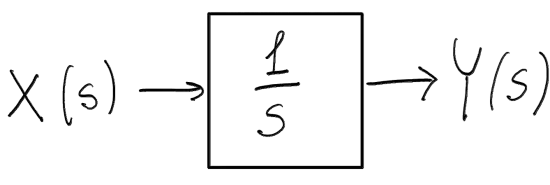Figure 3. A diagram of the analog integrator system.

We want to obtain a discrete system described by a discrete transfer function $G(z)$ that behaves like $\frac{1}{s}$. To this end, we observe the output of the continuous system at discrete time points $t = kT$, where $k \in \mathbb{Z}$ and $T$ is the sampling interval (inverse of the sampling rate).

$y(kT) = \int \limits_{0}^{kT} x(\tau) d\tau = \int \limits_{0}^{(k-1)T} x(\tau) d \tau + \int \limits_{(k-1)T}^{kT} x(\tau) d \tau \\= y((k-1)T) + \int \limits_{(k-1)T}^{kT} x(\tau) d \tau. \quad (6)$

We can now approximate the last integral in Equation 6 via the trapezoidal rule

$\int \limits_{(k-1)T}^{kT} x(\tau) d \tau \approx (x((k-1)T) + x(kT))T / 2. \quad (7)$

Inserting Equation 7 into Equation 6 yields

$y(kT) = y((k-1)T) + (x((k-1)T) + x(kT))T / 2. \quad (8)$

We now transform Equation 8 with the $z$-transformation

$Y(z) = z^{-1} Y(z) + (z^{-1} X(z) + X(z))T / 2. \quad (9)$

After rearranging Equation 9 we obtain the transfer function formulation

$Y(z) = \frac{T}{2}\frac{1 + z^{-1}}{1 - z^{-1}} X(z) = G(z) X(z). \quad (10)$

Since $G(z)$ was meant to approximate $\frac{1}{s}$, we can write

$\frac{1}{s} = G(z) = \frac{T}{2}\frac{1 + z^{-1}}{1 - z^{-1}}. \quad (11)$

which after inversion gives Equation 1, i.e., the bilinear transform. $\Box$

The bilinear transform can also be derived by representing a signal as a series of impulses, calculating its Laplace transform and $z$-transform, equating the two, and approximating the resulting $s$-to-$z$ mapping with a series expansion. But the integrator derivation seems more intuitive to me, especially the origin of the $\frac{2}{T}$ constant. What do you think? Let me know in the comments below the article. 😉

## Properties of the Bilinear Transform

In the following, we assume that $\omega_\text{a} = 2 \pi f_\text{a}$ (with $f_\text{a}$ in Hz) denotes the analog frequency in radians per second (value on the $j\omega$ axis of the $s$-plane) and $\omega_\text{d} = 2 \pi f_\text{d}$ (with $f_\text{d}$ in Hz) denotes the digital frequency in radians per second (corresponding to the angle of a point on the unit circle of the $z$-plane).

The bilinear transform has a number of useful properties [ParksBurrus87].

First of all, it is called bilinear because the numerator and the denominator are linear in $z$.

Second of all, the bilinear transform maps the left half-plane of the $s$-plane into the interior of of the unit circle in the $z$-plane. This is shown on Figure 4.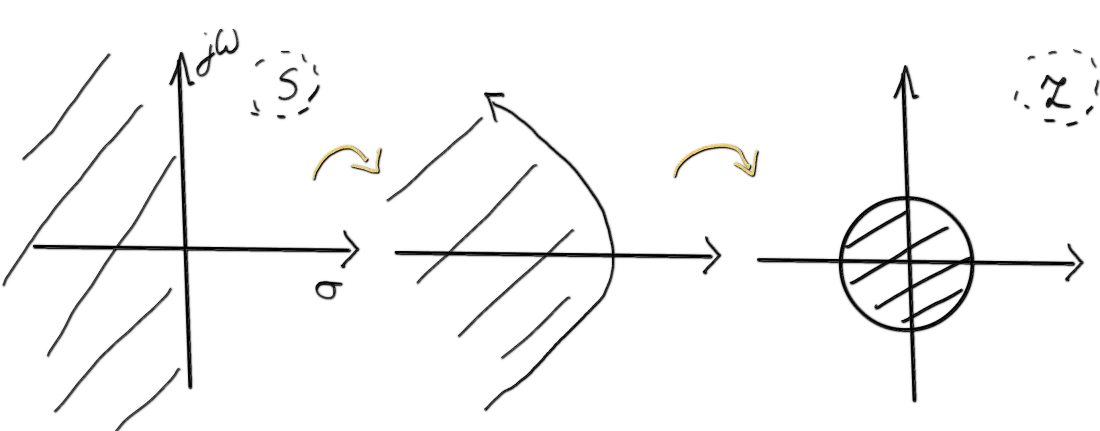Figure 4. Bilinear transform maps the left half-plane of the $s$-plane into the unit circle of the $z$-plane. The infinitely long analog frequency axis $j\omega$ becomes the finite-length circle. Axis bending in the middle is shown only for the visualization purposes: it is not what is meant by the mathematics.

As a consequence, the poles from the left half-plane of the $s$-plane are mapped to the poles within the unit circle of the $z$-plane. That means that stable analog filters are transformed into stable digital filters, what is a very desirable property in the context of musically useful parametric filters.

In the same way, the $j\omega_\text{a}$ analog frequency axis from the $s$-plane is mapped to the $z = e^{j\omega_\text{d}T}$ unit circle of the $z$-plane. That means that the analog system’s frequency response over an infinite domain gets squashed around a finite-length circle.

The above mappings mean that the bilinear transform is a nonlinear mapping of the phase and the frequency. The former results in the nonlinear phase of the resulting IIR filters [OppenheimSchafer10]. The latter manifests itself in the form of frequency warping as discussed later in the article.

Other properties of the bilinear transform are:

• the obtained digital filter has the same order as the analog prototype,
• optimality is preserved (e.g., maximally flat analog prototypes become maximally flat discrete filters),
• a cascade of systems transformed with the bilinear transform is equivalent to the bilinear transform of a cascade of these systems.

## Frequency Warping

The bilinear transform maps the full, infinite analog frequency axis to a finite-length discrete frequency axis (the unit circle). That results in frequency warping: “equal increments along the unit circle in the $z$ plane correspond to larger and larger bandwidths along the $j\omega_\text{a}$ axis in the $s$-plane” [Smith07].

The mathematical relation between the analog frequencies $\omega_\text{a}$ and digital frequencies $\omega_\text{d}$ can be found by evaluating the transform along the analog frequency axis and the digital frequency axis. In other words, we need to insert $s = j \omega_\text{a}$ and $z = e^{j\omega_\text{d} T}$ into Equation 1

$j \omega_\text{a} = \frac{2}{T}\frac{1 - e^{-j\omega_\text{d}T}}{1 + e^{-j\omega_\text{d}T}} = \frac{2}{T}\frac{e^{-j\omega_\text{d}T/2}(e^{j\omega_\text{d}T/2} - e^{-j\omega_\text{d}T/2})}{e^{-j\omega_\text{d}T/2}(e^{2j\omega_\text{d}T/2} + e^{-j\omega_\text{d}T/2})} \\= \frac{2}{T} \frac{j \sin(\omega_\text{d} T / 2)}{\cos(\omega_\text{d}T / 2)} = j \frac{2}{T} \tan(\omega_\text{d}T/2). \quad (12)$

Therefore,

$\omega_\text{a} = \frac{2}{T} \tan(\omega_\text{d}T/2). \quad (13)$

From Equation 13, we can obtain the inverse relation

$\omega_\text{d} = \frac{2}{T} \text{arctan}(\omega_\text{a} T / 2). \quad (14)$

The visualization of Equation 14 on Figure 5 shows what is frequency warping.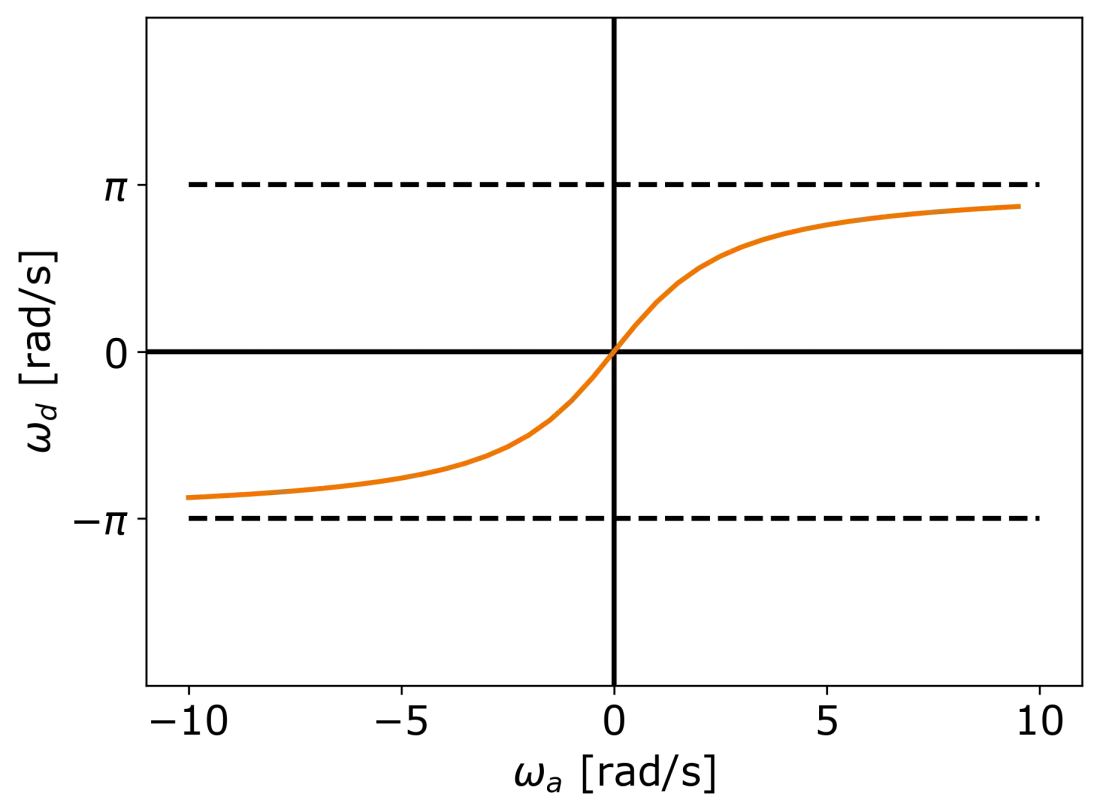Figure 5. Plot showing how analog frequencies are mapped to digital frequencies by the bilinear transform.

## Prewarping

In order to account for frequency warping of the bilinear transform in filter design, we need to perform the so-called prewarping. The prototype filter frequency scale must be prewarped so that after the bilinear transform the critical frequencies are in the correct places.

In other words, we want to have

$H_\text{a}(j \omega_\text{a0}) = H_\text{d}(\omega_\text{d0}) = H^*, \quad (15)$

where we choose $\omega_\text{a0}$ and $\omega_\text{d0}$ (desired critical ferquencies of the prototype and digital filters respectively).

This goal can be easily achieved by simply scaling the analog frequency axis with a proper scalar before the bilinear transform.

In the context of parametric filters, our critical frequency will typically be the cutoff frequency of a filter, because that’s the frequency for which we want to have a specific transfer function value. But let’s derive the general scaling.

### Prewarping Factor Derivation

Given critical frequencies $\omega_\text{a0}$ and $\omega_\text{d0}$, we want to find a scaling factor $K \in \mathbb{R}$ such that

$H_\text{a}(j \omega_\text{a0}) = H_\text{d}(\omega_\text{d0}) = H^*, \quad (16)$

and

$H_\text{d}(z) = H_\text{a}(K s) \forall z \in \mathbb{C}, \quad (17)$

where $s$ is given by Equation 1 (the bilinear transform).

From Equation 13, we know that without scaling by $K$

$\omega_\text{a0} = \frac{2}{T} \tan(\omega_\text{d0}T/2). \quad (18)$

Here $\omega_\text{a0}$ and $\omega_\text{d0}$ are coupled; we cannot change them independently. To change this, we introduce the scaling $K$ (Equation 17)

$\omega_\text{a0} = K \frac{2}{T} \tan(\omega_\text{d0}T/2). \quad (19)$

Solving for $K$

$K = \frac{\omega_\text{a0}}{\tan(\omega_\text{d0}T/2)} \frac{T}{2} \quad (20)$

allows us to independently change $\omega_\text{a0}$ and $\omega_\text{d0}$. $\Box$

So the bilinear transform combined with prewarping gives the following formula for $s$ substitution

$s = \frac{\omega_\text{a0}}{\tan(\omega_\text{d0}T/2)} \frac{1 - z^{-1}}{1 + z^{-1}}. \quad (21)$

### Cutoff Frequency from Prewarping

As you might recall from the analog prototype design tutorial, we design our analog filters to have the cutoff frequency equal to 1. Since thanks to prewarping, we can independently change $\omega_\text{a0}$ and $\omega_\text{d0}$, we can simply set $\omega_\text{a0} = 1$ and $\omega_\text{d0} = \omega_\text{dc}$ (cutoff frequency of the digital filter). Thus, Equation 21 (bilinear transform + prewarping) becomes

$s = \frac{1}{\tan(\omega_\text{d0} T/2)} \frac{1 - z^{-1}}{1 + z^{-1}}. \quad (22)$

So prewarping actally lets us set the cutoff frequency of the filter we design 🙂

## Example: Digitization of the Butterworth Low-Pass

Finally, let’s put to practice all that we’ve learned.

Let us digitize the analog prototype Butterworth low-pass of order 2 that we designed in the previous article.

It’s transfer function in the $s$-domain was

$H_a(s) = \frac{1}{s^2 + s \sqrt{2} + 1}. \quad (23)$

The cutoff frequency was set to 1.

To obtain a digital filter with the digital cutoff frequency equal to $\omega_\text{c}$ from Equation 23, we perform the bilinear transform with prewarping by inserting Equation 22 into Equation 23.

After very simple but tiresome derivations, we obtain

$H_2(z) = \frac{W^2 + 2W^2 z^{-1} + W^2z^{-2}}{1 + W \sqrt{2} + W^2 + 2(W^2 - 1)z^{-1} + (W^2 - W\sqrt{2} + 1)z^{-2}}, \quad (24)$

where $W = \tan(\omega_\text{c} T / 2)$ and $\omega_\text{c}$ is the desired cutoff frequency of the digital filter in radians per second. The above result matches the one in [Zölzer08].

We thus obtained a stable IIR filter of order 2 with controllable cutoff frequency. Yay!

### Efficiency

The filter in Equation 24 is efficient during processing but setting its cutoff frequency requires calculating the value of 5 coefficients (after normalization). That parameter-to-coefficient mapping is not efficient at all. Therefore, we need to turn to allpass-based filters… But that will be a topic of a different article. 😉

## Summary

In summary, in this article we learned that the bilinear transform is a great method that allows us to digitize analog prototype filters.

We learned how to derive the bilinear transform by approximating a continuous-time integrator. We learned the properties of the bilinear transform such as stability and optimality preservation. We also counteracted the frequency warping with prewarping.

We learned that with prewarping we can set the cutoff frequency of analog prototypes to 1 because prewarping alters it anyway.

Finally, we discretized the second-order analog Butterworth low-pass with the bilinear transform and prewarping and obtained an IIR filter.

Thanks for reading! If you have any questions, don’t hesitate to ask them in the comments below 🙂

## Bibiliography

Maximilian Schäfer, Music Processing - Synthesis lecture notes, Friedrich-Alexander-Universität Erlangen-Nürnberg, Winter Semester 2020/2021.

[Smith07] Julius O. Smith, Introduction to Digital Filters with Audio Applications, http://ccrma.stanford.edu/~jos/filters/, online book, 2007 edition, accessed November 26, 2021.

Links above may be affiliate links. That means that I may earn a commission if you decide to make a purchase. This does not incur any cost for you. Thank you.